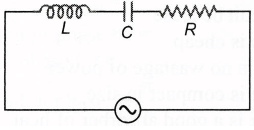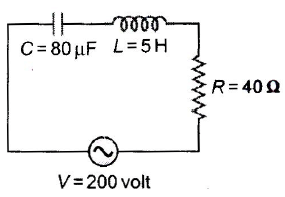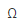Alternative Current MCQ Level - 1

# Alternative Current MCQ Level - 1

Test Description

## 30 Questions MCQ Test | Alternative Current MCQ Level - 1

Alternative Current MCQ Level - 1 for Class 12 2022 is part of Class 12 preparation. The Alternative Current MCQ Level - 1 questions and answers have been prepared according to the Class 12 exam syllabus.The Alternative Current MCQ Level - 1 MCQs are made for Class 12 2022 Exam. Find important definitions, questions, notes, meanings, examples, exercises, MCQs and online tests for Alternative Current MCQ Level - 1 below.
Solutions of Alternative Current MCQ Level - 1 questions in English are available as part of our course for Class 12 & Alternative Current MCQ Level - 1 solutions in Hindi for Class 12 course. Download more important topics, notes, lectures and mock test series for Class 12 Exam by signing up for free. Attempt Alternative Current MCQ Level - 1 | 30 questions in 60 minutes | Mock test for Class 12 preparation | Free important questions MCQ to study for Class 12 Exam | Download free PDF with solutions
 1 Crore+ students have signed up on EduRev. Have you?
Alternative Current MCQ Level - 1 - Question 1

### ln a choke coil, the reactance XL and resistance R are such that

Alternative Current MCQ Level - 1 - Question 2

### A100 V, AC source of frequency 500 Hz is connected to an L-C-R circuit with L = 8.1 mH, C = 12.5μF, R = 10W all connected in series as shown in figure. What is the quality factor of circuit?Alternative Current MCQ Level - 1 - Question 3

### In a circuit L, C and R are connected in series with an alternating voltage source of frequency f. The current leads the voltage by 45°. The value of C is

Alternative Current MCQ Level - 1 - Question 4

The natural frequency (ω0) of oscillations in L-C circuit is given by

Alternative Current MCQ Level - 1 - Question 5

In L-C-R series circuit the resonance condition in terms of capacitive reactance (XC) and inductive reactance (XL) is

Alternative Current MCQ Level - 1 - Question 6

Average power generated in an inductor connected to an AC source is

Alternative Current MCQ Level - 1 - Question 7

In L-C-R circuit if resistance increases, quality factor

Alternative Current MCQ Level - 1 - Question 8

An electric bulb has a rated power of 50 W at 100 V. If it is used on an AC source 200 V, 50 Hz, a choke has to be used in series with it. The should have an inductance of

Alternative Current MCQ Level - 1 - Question 9

From figure shown below a series L-C-R circuit connected to a variable frequency 200 V source. C = 80μF and R = 40W . Then the source frequency which drive the circuit at resonance isAlternative Current MCQ Level - 1 - Question 10

In an L-C-R series AC circuit, the voltage across each of components. L,C and R is 50 V. The voltage across the L-C combination will be

Alternative Current MCQ Level - 1 - Question 11

In an L-C-R circuit, capacitance is changed from C to 2C. For the resonant frequency to remain unchanged, the inductance should be changed from L to

Alternative Current MCQ Level - 1 - Question 12

Which of the following statement is incorrect?

Alternative Current MCQ Level - 1 - Question 13

The impedance of a circuit, when a resistance R and an Inductor of inductance L are connected in series in an AC circuit of frequency f, is

Alternative Current MCQ Level - 1 - Question 14

ln a series L-C-R circuit, resistance R = 10W and the impedance Z = 10W. The phase difference between the current and the voltage is.

Alternative Current MCQ Level - 1 - Question 15

A circuit consists of an inductance of 0.5 mH and a capacitor of 20μF. The frequency of the L-C oscillations is approximately

Alternative Current MCQ Level - 1 - Question 16

An inductor is connected to an AC source. When compared to voltage, the current in the lead wires

Alternative Current MCQ Level - 1 - Question 17

An inductance 1 H is connected in series with an AC source of 220 V and 50 Hz. The inductive reactance (in ohm) is

Alternative Current MCQ Level - 1 - Question 18

The power factor of an AC circuit having resistance R and inductance L (connected in series) and an angular velocity ω is

Alternative Current MCQ Level - 1 - Question 19

If the capacity of a condenser is 1 F, then its resistance in a DC circuit will be

Alternative Current MCQ Level - 1 - Question 20

A capacitor 50μF is connected to a supply of 220 V and angular frequency 50 rad s-1. The value of rms current in the circuit is

Alternative Current MCQ Level - 1 - Question 21

In a series L-C-R circuit the frequency of a 10 V AC voltage source is adjusted in such a fashion that the reactance of the inductor measures15and that of the capacitor11. If R = 3, the potential difference across the series combination of L and C will be

Alternative Current MCQ Level - 1 - Question 22

A circuit draws 330 W from a 110 V, 60 Hz AC line. The power factor is 0.6 and the current lags the voltage. The capacitance of a series capacitor that will result in a power factor of unity is equal to

Alternative Current MCQ Level - 1 - Question 23

In L-R circuit, resistance is 8and inductive reactanceis 6,  then impedance is

Alternative Current MCQ Level - 1 - Question 24

In L-C-R circuit, an alternating emf of angular frequency ω is applied then the total impedance will be

Alternative Current MCQ Level - 1 - Question 25

In AC circuit a resistance of Ris connected in series with an inductance L. If the phase difference between the current and voltage is 45°, the inductive reactance will be

Alternative Current MCQ Level - 1 - Question 26

The current which does not contribute to the power consumed in an AC circuit is called?

Alternative Current MCQ Level - 1 - Question 27

The power loss in AC circuit will be minimum when

Alternative Current MCQ Level - 1 - Question 28

In a series combination R = 300, L = 0.9 H, C = 2.0 μF, ω = 1000 rads-1, the impedance of the circuit is

Alternative Current MCQ Level - 1 - Question 29

For a series L-C-R circuit, the phase difference between current and voltage at the condition of resonance will be

Alternative Current MCQ Level - 1 - Question 30

In a L-R circuit of 3 mH inductance and 4resistance, emf E = 4 cos 1000tV is applied. The amplitude of emf is

 Use Code STAYHOME200 and get INR 200 additional OFF Use Coupon Code
Information about Alternative Current MCQ Level - 1 Page
In this test you can find the Exam questions for Alternative Current MCQ Level - 1 solved & explained in the simplest way possible. Besides giving Questions and answers for Alternative Current MCQ Level - 1, EduRev gives you an ample number of Online tests for practice